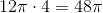## Example Questions

### Example Question #1 : How To Find The Ratio Of Diameter And Circumference

Letrepresent the area of a circle andrepresent its circumference. Which of the following equations expressesin terms of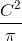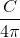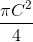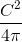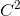Explanation:

The formula for the area of a circle is, and the formula for circumference is. If we solve for C in terms of r, we get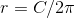.

We can then substitute this value of r into the formula for the area: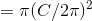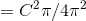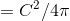### Example Question #1 : How To Find The Ratio Of Diameter And Circumference

A circle has a radius of. What is the ratio of its circumference to its area?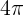Explanation:

1. Find the circumference: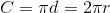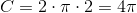2. Find the area: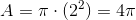3. Divide the circumference by the area: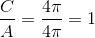### Example Question #2 : How To Find The Ratio Of Diameter And Circumference

How far must a racehorse run in alap race where the course is a circle with an area of?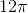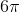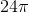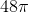Explanation:

To find the answer, we need to first find the circumference of the circle then mulitply that by 4 because the racehorse is running 4 laps.

We know that the area of the circle is. We can then find the radius.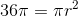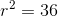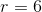Because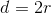, our diameter is 12.

We can now find the circumference.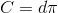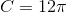Multiply the circumference by 4 (our racehorse is running 4 laps) to find the answer.